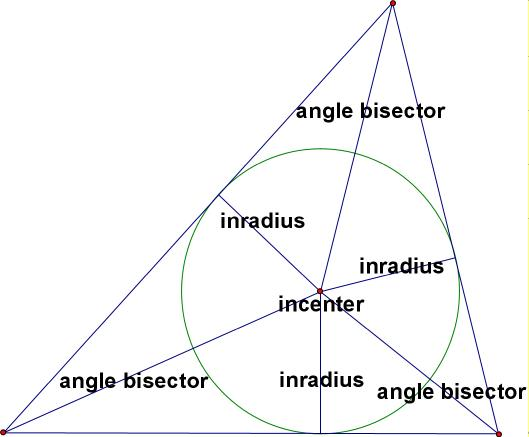# inscribed circle

Inscribed Circle
Incircle

The largest possible circle that can be drawn interior to a plane figure. For a polygon, a circle is not actually inscribed unless each side of the polygon is tangent to the circle.

Note: All triangles have inscribed circles, and so do all regular polygons. Most other polygons do not have inscribed circles. For a regular polygon, the inradius (the radius of the inscribed circle) is called the apothem.

Inscribed Circle of a Triangle